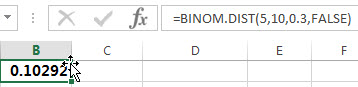# Excel BINOM.DIST Function

This post will guide you how to use the BINOM.DIST function with syntax and examples in Microsoft excel.

### Description

The Excel BINOM.DIST function returns the individual term binomial distribution probability. For example, you can use the BINOM.DIST function to calculate the probability that two of the next three babies born are male in Excel.

The BINOM.DIST function is a build-in function in Microsoft Excel and it is categorized as a Statistical Function.

The BINOM.DIST function is available in Excel 2016, Excel 2013, Excel 2010,  Excel 2011 for Mac.

### Syntax

The syntax of the BINOM.DIST function is as below:

`= BINOM.DIST(number_s, trials, probability_s, cumulative)`

Where the BINOM.DIST function arguments are:

• number_s -This is a required argument. The number of successes that you want to calculate the probability.
• Trials – This is a required argument.  The number of independent trials.
• probability_s – This is a required argument.  The probability of success for each trial.
• Cumulative – This is a required argument. It is a logical value that specifies the type of binomial distribution to be used. If the cumulative is TRUE, the BINOM.DIST function returns the cumulative distribution function; if it is equal to FALSE, the BINOM.DIST function returns the probability mass function.

Note:

• If number_s, trials, or probability_s argument is non-numeric, the BINOM.DIST functio will return #VALUE! Error.
• If number_s and trials argumetns are not integers and the BINOM.DIST function will truncate it.
• If number_s argument is smaller than 0 or greater than trials argument, the BINOM.DIST function will return the #NUM! Error.
• If probability_s is small than 0 or greater than 1, then the BINOM.DIST function will return the #NUM! Error.

### Excel BINOM.DIST Function Examples

The below examples will show you how to use Excel BINOM.DIST Function to get the binomial distribution probability for a given number of successes.

Example 1: to calculate the individual term binomial distribution probability for a given number of successes from a specified number of trials, using the following formula:
`=BINOM.DIST(5,10,0.3,FALSE)`### Related Functions

• Excel BINOMDIST function
The Excel BINOMDIST function returns the individual term binomial distribution probability. The syntax of the BINOMDIST function is as below:= BINOMDIST (number_s, trials, probability_s, cumulative).…
• Excel BETA.DIST function
The Excel BETA.DIST function can be used to calculate the cumulative beta distribution or beta probability density function.The syntax of the BETA.DIST function is as below:= BETA.DIST(x, alpha, beta, cumulative, [A], [B]).…